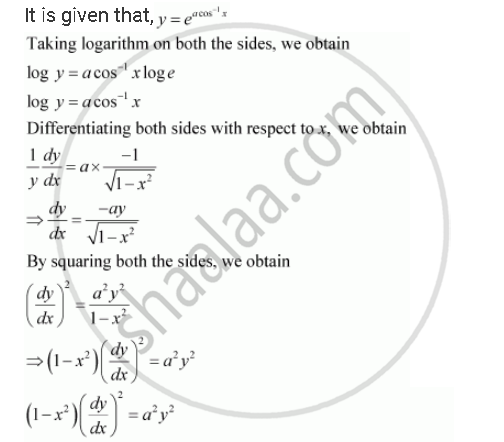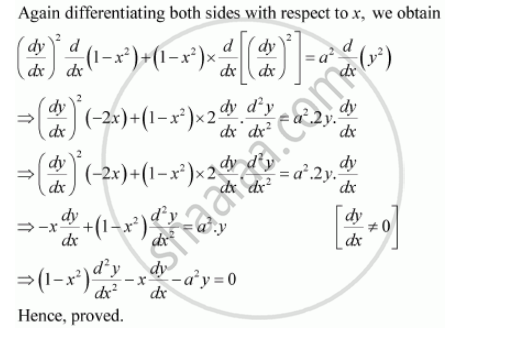Share

# If Y = E^(Acos^(-1)X), -1 <= X <= 1 Show that (1- X^2) (D^2y)/(Dx^2) -x Dy/Dx - A^2y = 0 - CBSE (Science) Class 12 - Mathematics

#### Question

if y = e^(acos^(-1)x), -1 <= x <= 1 show that (1- x^2) (d^2y)/(dx^2) -x dy/dx - a^2y = 0

#### SolutionIs there an error in this question or solution?

#### APPEARS IN

NCERT Solution for Mathematics Textbook for Class 12 (2018 to Current)
Chapter 5: Continuity and Differentiability
Q: 23 | Page no. 192

#### Video TutorialsVIEW ALL 

Solution If Y = E^(Acos^(-1)X), -1 <= X <= 1 Show that (1- X^2) (D^2y)/(Dx^2) -x Dy/Dx - A^2y = 0 Concept: Logarithmic Differentiation.
S